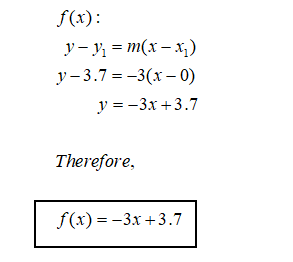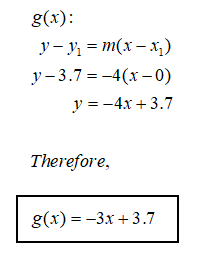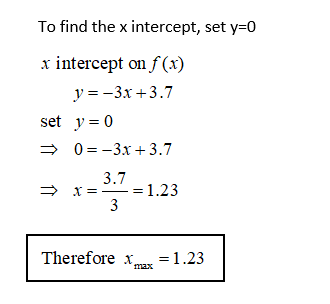# This is not a drill! The rocket set off by Crashcon industries has developed a catastrophic defect; destroying the thrusters and sending the rocket plummeting to earth. Our detection center at (0,0) has determined the height of the rocket's trajectory to be (0,3.7) miles. It has been comfirmed that the rocket is traveling in a linear path. But we have not been able to determine a definitive slope- we know that the slope is between -3 and -4. We need to use this information to determine a region of potential impact.Using the same point (0,3.7), find two linear functions. f(x) with a slope of -3 and g(x) with a slope of -4Find the x-intercept of both functions; these are the maximum (xmax) and minimum (xmin) points of impact.In set notation establish a region of potential impact                       {x|xmin  < x < xmax }

Question
12 views

This is not a drill! The rocket set off by Crashcon industries has developed a catastrophic defect; destroying the thrusters and sending the rocket plummeting to earth. Our detection center at (0,0) has determined the height of the rocket's trajectory to be (0,3.7) miles. It has been comfirmed that the rocket is traveling in a linear path. But we have not been able to determine a definitive slope- we know that the slope is between -3 and -4. We need to use this information to determine a region of potential impact.

• Using the same point (0,3.7), find two linear functions. f(x) with a slope of -3 and g(x) with a slope of -4
• Find the x-intercept of both functions; these are the maximum (xmax) and minimum (xmin) points of impact.
• In set notation establish a region of potential impact                       {x|xmin  < x < xmax }
check_circle

Step 1

Given (x,y) and slope, we can use the slope point form and determine the functions.Step 2Step 3...

### Want to see the full answer?

See Solution

#### Want to see this answer and more?

Solutions are written by subject experts who are available 24/7. Questions are typically answered within 1 hour.*

See Solution
*Response times may vary by subject and question.
Tagged in

### Calculus